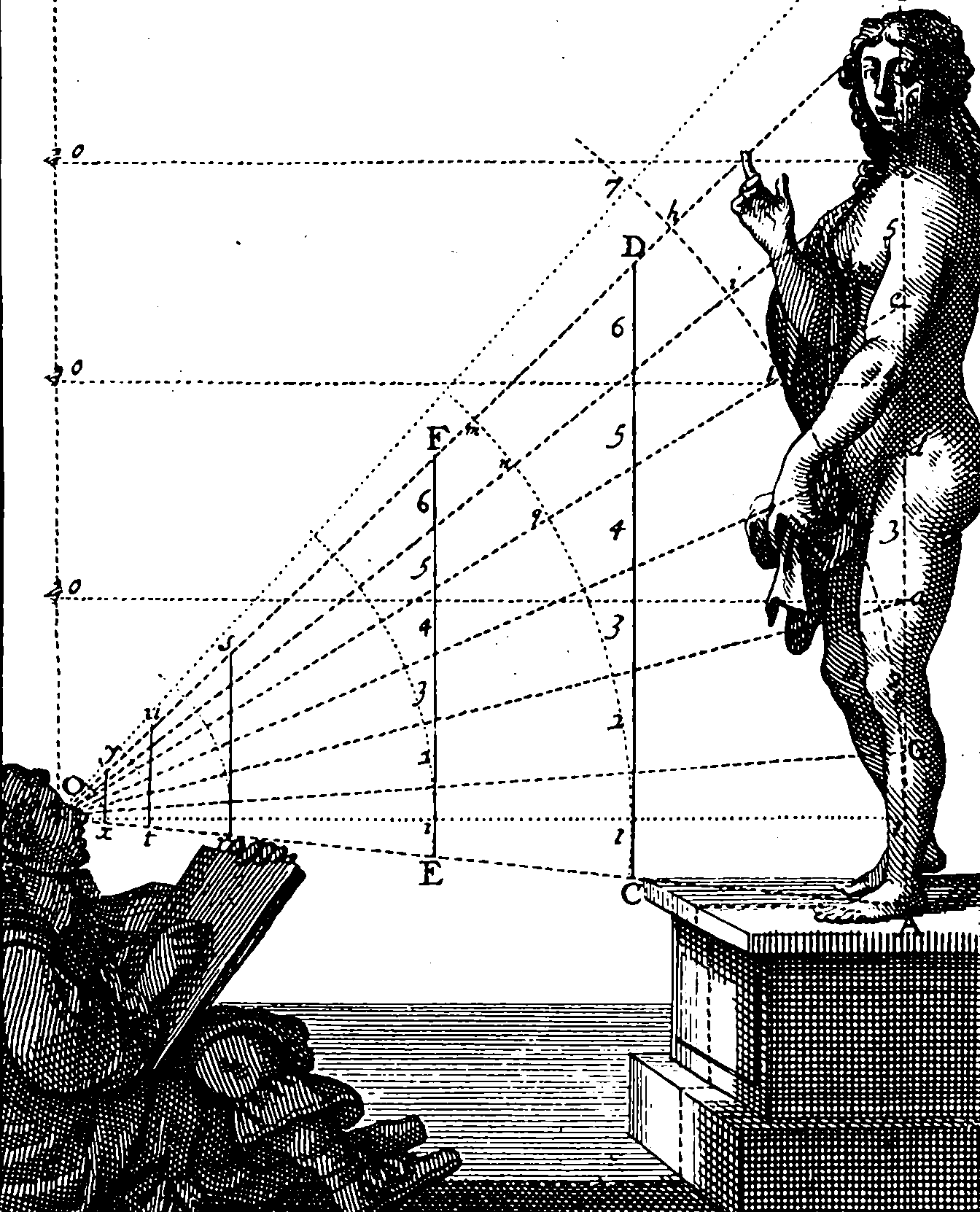# Warping and registration problems

## Matching up of bumps and wibbles in stretchy thingsVarious notes on a.e. continuous monotonic changes of index to align two process, and estimation thereof.

Warping, registration problems. Especially interesting for functional data analysis, and analysis/resynthesis. A deterministic problem related to statistical changes of time.

General setup:

You have two functions $$\phi,\psi$$, which we assume for now are both $$\mathbb{R}^+\rightarrow \mathbb{R}$$ and you wish to align them with respect to some pointwise loss, $$\ell$$ and some class of permissable class $$\mathcal{F}$$ of warping functions $$\mathbb{R}^+\rightarrow\mathbb{R}^+$$, and you wish to do this over some interval $$D$$.

This means that you wish to find

$\argmin_{f\in \mathcal{F}} \int_D(\ell(\phi(t), \psi(f(t))))dt$

## Affine warps of a real function

Image/sound alignment problems are an interesting special case where we usually want affine warps, i.e. $$f(t)=at+b) for some constants \(a,b.$$ Here the problem is mathematically not so complex, but in practice it can become computationally intractable; In particular, let’s say your function is interpolated from data, i.e. we observe $$\phi(k), k=1,2,3\dots,N,$$ and $$\phi(k), k=1,2,3\dots,N.$$ and construct a nice interpolant $$\hat{\phi}$$ Leaving aside statistical questions about how accurate this is, which is a whole other story, how computationally feasible is this to check for alignment with some other ?

Let’s say we construct our interpolant from some mathematically justified basis, such as the sinc basis:

$\hat{\phi(t)}=\sum_{k=1}^N \sinc (t-k)\phi(k)$

So that’s nicely linear and trivial to calculate. The loss integral then is

\begin{aligned} \int_D\ell(\hat{\phi}(t), \hat{\psi}(f(t)))dt = \int_D\ell(\sum_{k=1}^N \sinc (t-k)\phi(k), \sum_{k=1}^N \sinc (f(t-k))\psi(k), ) \end{aligned}

We might try to approximate this integral by sampling it at some lattice or other subset, or we might have analytic forms for the integrals of the terms or whatever.

Either way, though, this basis expansion is expensive, requiring $$\mathcal{O}(N^2)$$ evaluations, which is inconvenient.

If we’ve smoothed our data somewhat and have a spline basis, this can be better, although then we still have a smoothing problem to solve. As an irritating technical detail, FITPACK — which is the Fortan spline library which seems to be everywhere — has, AFAICT, no function to take the product of two splines or to do an affine transform of the coordinate, or take derivatives with respect to a coordinate transform etc, so despite the fact that everything is simple in principle, so you have to do an enormous amount of book-keeping to do this.

## General linear transformsSay you are in 2d, or 3d and now you wish to register two objects by finding an optimal linear transform…

## Smooth nonlinear transforms

Dynamic Time Warping. (Does it work in multiple axes?)

soft-dtw

To compute DTW, one typically solves a minimal-cost alignment problem between two time series using dynamic programming. Our work takes advantage of a smoothed formulation of DTW, called soft-DTW, that computes the soft-minimum of all alignment costs. We show in this paper that soft-DTW is a differentiable loss function, and that both its value and gradient can be computed with quadratic time/space complexity (DTW has quadratic time but linear space complexity)

🏗

## Other warps

Does the CTC warp-robust loss function fit here? I think it’s for discretely-indexed language data, which is not quite the kind of continuous form I’m worried about.

CTC:

Connectionist Temporal Classification is a loss function useful for performing supervised learning on sequence data, without needing an alignment between input data and labels. For example, CTC can be used to train end-to-end systems for speech recognition, which is how we have been using it at Baidu’s Silicon Valley AI Lab.

## Warping of point processes

A special interest of mine, at the intersection of functional data analysis, point processes and warping.

Though the study of multiple realisations of point processes has been considered prior to the emergence of FDA …, treating realisations of point processes as individual data objects within a functional data analysis context is a more recent development offering important advantages; a key paper is that of (also see Chiou and Müller …). Such data may be an object of interest in themselves (see, e.g., Arribas-Gil and Müller (2014);Wu, Müller, and Zhang (2013),…) but may also arise as landmark data in an otherwise classical functional data analysis (see, e.g., …, Arribas-Gil and Müller (2014)). The recent surge of interest is exemplified in an upcoming discussion paper by …, whose discussion documents early progress and challenges in the field.

## Warping to achieve stationarity

See kernel warping.

### No comments yet. Why not leave one?

GitHub-flavored Markdown & a sane subset of HTML is supported.